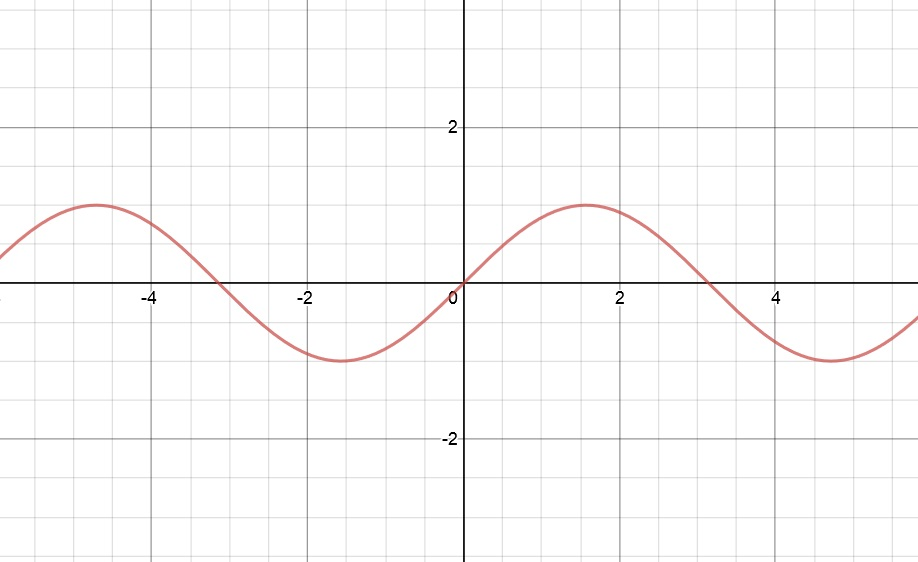James Dale

2022-01-13

Determine the end behavior of the following transcendental functions by analyzing appropriate limits. Then provide a simple sketch of the associated graph, showing asymptotes if they exist.
$f\left(x\right)=\mathrm{sin}x$redhotdevil13l3

Step 1
Substitute for x
$\underset{x\to \mathrm{\infty }}{lim}=\underset{x\to \mathrm{\infty }}{lim}\mathrm{sin}\left(\mathrm{\infty }\right)$
Simplify
$=Undefined$
Substitute for x
$\underset{x\to -\mathrm{\infty }}{lim}f\left(x\right)=\underset{x\to -\mathrm{\infty }}{lim}\mathrm{sin}\left(-\mathrm{\infty }\right)$
Simplify
$=Undefined$
Step 2
Sine is defined on entire domain so we have no vertical asymptotes.
For slant asymptotes, we check the lines.
Using formula $\underset{x\to ±\mathrm{\infty }}{lim}\frac{f\left(x\right)}{x}=k$ where k represents slope of our line (slant asymptote).
Table limit
$\underset{x\to \mathrm{\infty }}{lim}\frac{f\left(x\right)}{x}=\underset{x\to \mathrm{\infty }}{lim}\frac{\mathrm{sin}\left(x\right)}{x}$
Simplify
$=0$
Therefore, there is no slant asymptote.Do you have a similar question?